# Polynomials - Olympiad Level MCQ, Class 10 Mathematics

## 35 Questions MCQ Test Olympiad Preparation for Class 10 | Polynomials - Olympiad Level MCQ, Class 10 Mathematics

Description
Attempt Polynomials - Olympiad Level MCQ, Class 10 Mathematics | 35 questions in 35 minutes | Mock test for Class 10 preparation | Free important questions MCQ to study Olympiad Preparation for Class 10 for Class 10 Exam | Download free PDF with solutions
QUESTION: 1

### If α,β and γ are the zeros of the polynomial 2x3 – 6x2 – 4x + 30, then the value of (αβ + βγ + γα) is

Solution:

We have a 3 degree polynomial here of form
ax3 + bx2 + cx +d = 0
Polynomial p(x) = 2x3 – 6x2 – 4x + 30

The roots of this polynomial are α, β, γ
We have formula for products of zeroes taking 2 at a time = αβ + βγ + αγ = c/a
αβ + βγ + αγ = -4/2 = -2

QUESTION: 2

### The quadratic polynomial whose sum of zeroes is 3 and product of zeroes is –2 is :

Solution:

Sum of zeroes = 3

Product of zeroes = -2

Let the quadratic polynomial be ax2 + bx + c

Sum of zeroes = 3

-b/a = 3/1

So a = 1, b = -3

Product of zeroes = -2

c/a = -2/1

So a = 1, c = -2

Hence the  quadratic polynomial will be   x2 - 3x - 2

QUESTION: 3

### If x + 2 is a factor of x3 – 2ax2 + 16, then value of a is

Solution:

Given ,g(x)=x+2=0
x=-2

P(x)=x3-2ax2+16
p(-2)=(-23) -2 × a × (-22)+16
=-8 - 8a +16
=8 - 8a
=>-8a = -8
=>a=1

QUESTION: 4

If α,β and γ are the zeros of the polynomial f(x) = x3 + px2 – pqrx + r, then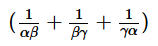=

Solution:
QUESTION: 5

If the parabola f(x) = ax2 + bx + c passes through the points (–1, 12), (0, 5) and (2, –3), the value of a + b + c is –

Solution:

f(x)=ax2+bx+c

It is given that f(0)=5, f(−1)=12 and f(2)=−3

f(0)=c=5 ... (i)

f(−1)=a−b+c=12

∴a−b=12−c

∵c=5

∴a−b=12−5 =7 ... (ii)

f(2)=4a+2b+c=−3

⇒4a+2b=−3−c

as c=5

⇒4a+2b=−8

⇒2a+b=−4 ... (iii)

Solving (ii) and (iii), we get

a=1

b=−6

Hence, a=1,b=−6,c=5

Thus, a+b+c =1−6+5 =0.

QUESTION: 6

If a, b are the zeros of f(x) = x2 + px + 1 and c, d are the zeros of f(x) = x2 + qx + 1 the value of E = (a – c) (b – c) (a + d) (b + d) is –

Solution:

The given polynomials are

f(x) = x² + px + 1

g(x) = x² + qx + 1

Since a, b are the zeroes of f(x),

a + b = - p ..... (1)

ab = 1 ..... (2)

Since c, d are the zeroes of g(x),

c + d = - q ..... (3)

cd = 1 ..... (4)

Now, E = (a - c) (b - c) (a + d) (b + d)

= {ab - (a + b)c + c²} {ab + (a + b)d + d²}

= (1 + pc + c²) (1 - pd + d²) [by (1) & (2)]

= 1 - pd + d² + pc - p²cd + pcd² + c² - pc²d + c²d²

= 1 - pd + d² + pc - p²(cd) + p(cd)d + c² - pc(cd) + (cd)²

= 1 - pd + d² + pc - p² + pd + c² - pc + 1 [by (4)]

= 2 + c² + d² - p²

= 2 + (c + d)² - 2(cd) - p²

= 2 + (- q)² - 2 - p² [by (3) & (4)]

= q² - p²

QUESTION: 7

If α,β are zeros of ax+ bx + c then zeros of a3x2 + abcx + c3 are –

Solution: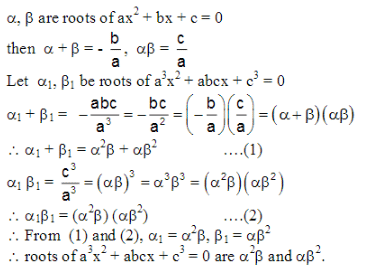QUESTION: 8

Let α,β be the zeros of the polynomial x2 – px + r and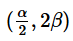be the zeros of x2 – qx + r. Then the value
of r is –

Solution:

Since a and b are roots of  x2 – px + r = 0
We can say a+b=p....say equation 1 and ab=r
Also a/2 and 2b are roots of  x2 – qx + r
So, a/2 +2b=q ..... say equation 2 and a/2*2b=ab=r
2(equation1)-equation 2 = 2a -a/2 +2b-2b = 2p-q
so 3a/2=2p-q
a=2(2p-q)/3
Substituting in equation 1
We get b=p-4p/3+2q/3=(2q-p)/3
So we know ab=r=2(2p-q)(2q-p)/9

QUESTION: 9

When x200 + 1 is divided by x2 + 1, the remainder is equal to –

Solution:

When x200 + 1 is divided by x2 + 1, the remainder is equal to

x²⁰⁰ + 1 =  x²⁰⁰ + (x¹⁹⁸ - x¹⁹⁸ - x¹⁹⁶ + x¹⁹⁶ + x¹⁹⁴  - x¹⁹⁴ - x¹⁹² + x¹⁹² +x¹⁹⁰ - x¹⁹⁰ +..................-x⁴ + x⁴ + x² - x² - 1 + 1 )+ 1

=> x²⁰⁰ + 1  = x²⁰⁰ + x¹⁹⁸ - x¹⁹⁸ - x¹⁹⁶ + x¹⁹⁶ + x¹⁹⁴  - x¹⁹⁴ - x¹⁹² + x¹⁹² +x¹⁹⁰ - x¹⁹⁰ +..................-x⁴ + x⁴ + x² - x² - 1 + 1 + 1

=> x²⁰⁰ + 1  = x¹⁹⁸(x² + 1) - x¹⁹⁶(x² + 1) + x¹⁹⁴(x² + 1)  - x¹⁹²(x² +1) + x¹⁹⁰(x² +1) - x¹⁸⁸(x²+1) +..................+ x²(x² + 1) - (x² + 1) + 1 + 1

=>  x²⁰⁰ + 1  = x¹⁹⁸(x² + 1) - x¹⁹⁶(x² + 1) + x¹⁹⁴(x² + 1)  - x¹⁹²(x² +1) + x¹⁹⁰(x² +1) - x¹⁸⁸(x²+1) +..................+ x²(x² + 1) - 1.(x² + 1) + 2

=>  x²⁰⁰ + 1  = (x² + 1)(x¹⁹⁸- x¹⁹⁶ + x¹⁹⁴ - x¹⁹² + x¹⁹⁰ - x¹⁸⁸+..................+ x² - 1) + 2

=> when x²⁰⁰ + 1 is divided by x² + 1  the reminder = 2

QUESTION: 10

If a (p + q)2 + 2bpq + c = 0 and also a(q + r)2 + 2bqr + c = 0 then pr is equal to –

Solution:

A*(p+q)^2 + 2bpq + c = 0 and a*(p+r)^2 +2bpr + c = 0
=> aq^2 + 2p(a+b)q + ap^2 + c = 0
and ar^2 + 2p(a+b)r + ap^2 + c = 0

=> q and r are the roots of the equation
ax^2 + 2p(a+b)x + ap^2 + c = 0
=> qr = (ap^2 + c) / a.

QUESTION: 11

If a,b and c are not all equal and α and β be the zeros of the polynomial ax2 + bx + c, then value of (1 + α + α2) (1 + β + β2)  is :

Solution:
QUESTION: 12

Two complex numbers α and β are such that α + β = 2 and α4 + β4 = 272, then the polynomial whose zeros
are α and β is –

Solution:
QUESTION: 13

If 2 and 3 are the zeros of f(x) = 2x3 + mx2 – 13x + n, then the values of m and n are respectively –

Solution:
QUESTION: 14

If α,β are the zeros of the polynomial 6x2 + 6px + p2, then the polynomial whose zeros are (α + β)2 and (α - β)2 is

Solution:
QUESTION: 15

If c, d are zeros of x2 – 10ax – 11b and a, b are zeros of x2 – 10cx – 11d, then value of a + b + c + d is –

Solution:
QUESTION: 16

If the ratio of the roots of polynomial x2 + bx + c is the same as that of the ratio of the roots of x2 + qx + r, then –

Solution: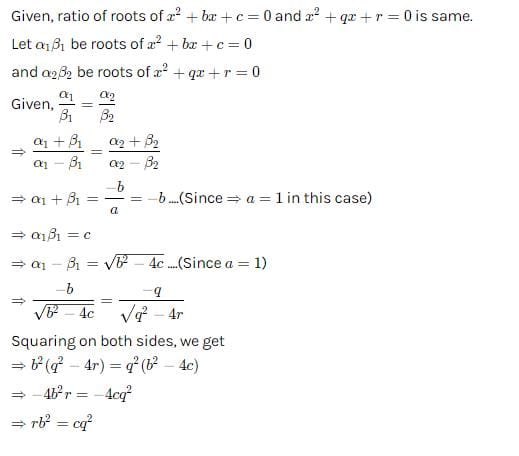QUESTION: 17

If the roots of the polynomial ax2 + bx + c are of the form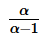and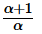then the value of (a + b + c)2 is–

Solution:
QUESTION: 18

If α, β and γ are the zeros of the polynomial x3 + a0x2 + a1x + a2, then (1 – α2) (1 – β2) (1 – γ2) is

Solution:
QUESTION: 19

If α,β,γ are the zeros of the polynomial x3 – 3x + 11, then the polynomial whose zeros are (α + β), (β + γ) and
(γ + α) is –

Solution:
QUESTION: 20

If α,β,γ are such that α + β + γ = 2, α2 + β2 + γ2 = 6, α3 + β3 + γ3 = 8, then α4 + β4 + γ4 is equal to–

Solution:

(α+β+γ)2222+2(αβ+βγ+αγ)

⇒4=6+2(αβ+βγ+αγ)

⇒αβ+βγ+αγ=−1

333−3αβγ)=(α+β+γ)(α222−αβ−βγ−αγ)

⇒8−3αβγ=2(6+1)

⇒αβγ=−2

222)2444+2(α2β22γ22γ2)

⇒α434+2[(αβ+βγ+αγ)2−2αβγ(α+β+γ)]

⇒36=α444+2[(−1)2−2(−2(2)]

⇒α444=36−18

⇒18

Hence (C) is the correct answer

QUESTION: 21

If α,β are the roots of ax2 + bx + c and α + k, β + k are the roots of px2 + qx + r, then k =

Solution:
QUESTION: 22

If α,β are the roots of the polynomial x2 – px + q, then the quadratic polynomial, the roots of which are (α2– β2) (α3 – β3) and (α3β2 + α2β3) :

Solution:

If α,β are the roots of the polynomial x2 - px + q.

α + β = p and αβ = q

if the roots are (α22)(α3 - β3) and (α3β2 + α2β3)

= (α22)(α3 - β3) + (α3β2 + α2β3)

= (α +β)(α -β)2(α2 + αβ+ β2) + α2β2(α + β).

= (α +β)[ (α +β)2 - 4αβ ] [ (α +β)2 - αβ ] + α2β2(α + β).

= p( p2 - 4q)( p2 - q) + q2p

= p( p4 - p2q -4p2q + 4q2) + pq2

= p5 - 5p3q + 5pq2 .

⇒ (α22)(α3 - β3)(α3β2 + α2β3) = (p6q2-5p4q3+4p2q4)

∴ x2- (p5 - 5p3q + 5pq2 ) x + ( p6q2 - 5p4q3 + 4p2q4 ) = 0

QUESTION: 23

The condition that x3 – ax2 + bx – c = 0 may have two of the roots equal to each other but of opposite signs is:

Solution: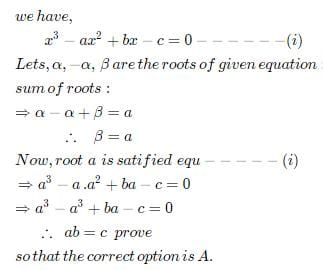QUESTION: 24

If the roots of polynomial x2 + bx + ac are α,β and roots of the polynomial x2 + ax + bc are α,γ then the values of α,β,γ respectively are –

Solution:

α+β=−b
αβ=ac
Similarly,
α+γ=−a
αγ=bc
Now α is the common root.
Therefore, from the equations,
αβ=ac and αγ=bc
We get the common root α=c
Therefore
β=a and γ=b

Since α+β=−b
Hence
c+a=−b
a+b+c=0

QUESTION: 25

If one zero of the polynomial ax2 + bx + c is positive and the other negative then (a,b,c εR, a = 0)

Solution:

if α and β are two roots of equation ax2+bx+c=0

product of roots α*β = c/a

now if one root is negative and other positive, then product of the roots should be negative.

i.e c/a is negative which means both a and c are of opposite signs.

QUESTION: 26

If α,β are the zeros of the polynomial x2 – px + q, then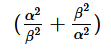is equal to –

Solution:
QUESTION: 27

If α,β are the zeros of the polynomial x2 – px + 36 and α2 + β2 = 9, then p =

Solution:

sum of roots α+β= -b/a = p

product of roots αβ = c/a= 36

α22= 9

α2+2αβ - 2αβ = 9

(α+β)- 2αβ = 9

p2= 9 + 2*36

p= 81

p = 9

QUESTION: 28

If α,β are zeros of ax2 + bx + c, ac =  0, then zeros of cx2 + bx + a are –

Solution:

α+β = -b/a    (eq1)    αβ = c/a  (eq 2)

let the second equation cx2 + bx + a has roots x and y

x+y = -b/c   (eq 3)   xy = a/c (eq 4)

divide eq 1 by eq 3

α+β / x+y = (-b/a) / (-b/c)

= c/a

= αβ

α+β / αβ = x+y

x+y = 1/α + 1/β

QUESTION: 29

A real number is said to be algebraic if it satisfies a polynomial equation with integral coefficients. Which of the following numbers is not algebraic :

Solution:

transcendental number is a complex number that is not algebraic—that is, not a root (i.e., solution) of a nonzero polynomial equation with integer or equivalently rational coefficients. The most popular transcendental numbers are π and e

QUESTION: 30

The bi-quadraic polynomial whose zeros are 1, 2, 4/3, - 1 is :

Solution:

As the roots are 1,2,4/3,-1

Therefore the equation will be

(x-1)(x-2)(x-4/3)(x+1)

If we will solve this thing then we will get

3x4 – 10x3 + 5x2 + 10x – 8

QUESTION: 31

The cubic polynomials whose zeros are 4,3/2 and – 2 is :

Solution:

roots are 4,3/2 and – 2 so we can form equation as

(x-4)(x-3/2)(x-2)=0

which will give 2x3 – 7x2 – 10x + 24

QUESTION: 32

If the sum of zeros of the polynomial p(x) = kx3 – 5x2 – 11x – 3 is 2, then k is equal to :

Solution:

Let α,β,γ be the zeroes of given polynomial p(x)......
then,
α+β+γ= -b/a = 2   (given)
=> 2 = -(-5)/k
=> k =5/2

QUESTION: 33

If f(x) = 4x3 – 6x2 + 5x – 1 and α, β and γ are its zeros, then αβγ  =

Solution:

for a cubic equation product of roots is α,β,γ = -d/a

= -(-1)/4   = 1/4

QUESTION: 34

Consider f(x) = 8x4 – 2x2 + 6x – 5 and α,β,γ,δ are it's zeros then α + β + γ + δ =

Solution:

for a equation of 4 roots ax4+bx3+cx2+dx+e=0

sum of roots is -b/a

in our equation b = 0 (since there is no term of x3)

so sum of roots is -b/a=0

QUESTION: 35

If x2 – ax + b = 0 and x2 – px + q = 0 have a root in common and the second equation has equal roots, then –

Solution:

x2 – ax + b = 0 and x2 – px + q = 0 are the equations

second equation has two equal roots let the roots be k

2k = p => k = p/2   and k2=q

now both equations has one common root k

for first equation k+ l = a => l = a- p/2      and kl = b

put k and l

p/2 (a-p/2) = b

ap/2 - p2/4 = b

ap/2 = k2+b

ap/2 = q+bUse Code STAYHOME200 and get INR 200 additional OFF Use Coupon Code